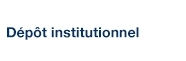# On Lagerstrom’s Model of Slow Incompressible Viscous Flow

Hunter, C.; Tajdari, M. et Boyer, S. D. (1990). « On Lagerstrom’s Model of Slow Incompressible Viscous Flow ». SIAM Journal on Applied Mathematics, 50(1), pp. 48-63.

Fichier(s) associé(s) à ce document :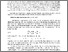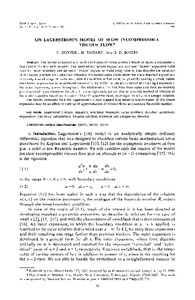Prévisualisation
PDF
Télécharger (1MB)

## Résumé

The model discussed is a nonlinear boundary value problem which contains a parameter $\varepsilon$ that models the Reynolds number. The matched asymptotic expansions, an inner “Stokes” expansion valid near the inner boundary and an outer “Oseen” expansion valid away from it, that describe the solutions of the model problem for $\varepsilon$ small are extended. Numerical calculations show that these matched expansions have only a small range of usefulness, with the addition of further terms generally causing a worse, rather than better, approximation at moderate values of $\varepsilon$. Far better results are achieved when a single expansion, the outer expansion, is used throughout. The additional terms that have been calculated then consistently give improved approximations for all $\varepsilon$. It is also rigorously proved that an iterative method of solution of the model equation based on the outer “Oseen” approximation, converges for all $\varepsilon$ to a unique solution. The results presented here for Lagerstrom’s model suggest that iterative improvement of the Oseen expansion may be an effective method of approximation of viscous flows at moderate Reynolds number.

Type: Article de revue scientifique Lagerstrom's model equation, nonlinear boundary value problem, matched asymptotic expansions, numerical calculations, iterative solution, existence and uniqueness theorem Faculté des sciences > Département de mathématiques Steven P. Boyer 18 avr. 2016 17:19 27 avr. 2016 18:14 http://archipel.uqam.ca/id/eprint/8173

## Statistiques

Voir les statistiques sur cinq ans...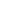RECHERCHER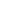PARCOURIR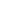LIBRE ACCÈS